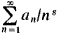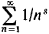# Dirichlet Series

Also found in: Wikipedia.

## Dirichlet series

[‚dē·rē′klā ‚sir·ēz]
(mathematics)
A series whose n th term is a complex number divided by n to the z th power.

## Dirichlet Series

(named for P. G. L. Dirichlet), series of the formwhere the an are constants and s = σ + it is a complex variable. For example, the seriesrepresents the zeta function for σ > 1. The theory of Dirichlet series originally arose under the strong influence of analytic number theory. Eventually it developed into an extensive branch in the theory of analytic functions.

References in periodicals archive ?
Among the topics are quadratic points of classical modular curves, p-adic point counting on singular super-elliptic curves, a vanishing criterion for Dirichlet series with periodic coefficients, the Sato-Tate conjecture for a Picard curve with a complex multiplication, arithmetic twists with abelian extensions, and transcendental numbers with special values of Dirichlet series.
Nakajima, A new expression for the product of the two Dirichlet series.
The kernel function can be expressed in Dirichlet Series form :
This requires the associated multiplicative function, say f(n), to have special enough properties that the associated Dirichlet series may be analytically continued.
Gunnells, Metaplectic ice, in Multiple Dirichlet Series, L-Functions, and Automorphic Forms, D.
It is easily seen the Dirichlet series is absolutely convergent for Rs = [sigma] > 1/6.
Widder (1898-1990) drew material from a course of lectures that he gave now and then at Harvard on Dirichlet series and Laplace integrals, to explain the Laplace transform for students who have a fundamental knowledge of analysis, though proofs are included for those with only a little such knowledge.
alpha]] ([alpha] [member of] C) forms an Abelian group with the Dirichlet series multiplication followed by a number of applications.
However, the formula may be used to construct twin prime Dirichlet series.
This article presents a new algorithm for nonharmonic signal analysis using Dirichlet series
We attach a Dirichlet series D(s,f) [Mathematical Expression Omitted] by (2.
s] and essentially those Dirichlet series where one might expect a Riemann hypothesis.

Site: Follow: Share:
Open / Close